# Functions in C Programming

## Introduction

Suppose we have to write a program to input two numbers and perform arithmetic operation that user asks for. User can ask for any of the arithmetic operations like addition, subtraction, multiplication or division. How will we write a program? We will be writing single C program with main function, where we will accept two numbers and operation to be performed. Depending on the operation that user has entered we will have if condition where we will add / subtract/ multiply / divide the numbers. These are the straight forward steps for this simple requirement. Imagine these operations on two numbers increases to addition of more numbers or array of numbers. Then the code within each ‘if’ statement will also increase. That means as the requirement changes or gets complex, the code also gets increased and becomes complex. This in turn reduces the readability of the code too. What will happen if we perform these set of operations within a named block and call this block whenever it is required? It increases the readability of code as well as makes the user to understand it easily.

For example, the same arithmetic operations for array of numbers are created in different functions like addition (), subtraction (), multiplication () and division () and in the main function if we call these functions in the ‘if’ statement of the operation, then the code looks simpler and easier to understand. The logic used for these operations are of less important here. If we need to know the logic then we can check the respective function. Suppose there is some additional requirement while dividing to check if the divisor is zero. Then we need not traverse the whole main function till we get division operation code. Rather we can directly fetch the division () function and add the new set of code to check of zero. Thus creating the function makes the addition of new requirement also simpler. Hence in C functions are vastly used.

In C everything is written within a function. The program itself begin from the main () function. As the name suggests, it is the main function in any program, from which the execution of the code starts. Other requirements of the user are then divided into multiple functions and are called from the main function. Any other function can also call same or other function. In short C is not complete without functions!

A function can accept one or more inputs, perform some operation on them, and may or may not return the output to the calling function. It can be called from any function, any number of times. That means a function is a named reusable block of code in the program.

## Types of Function

In C, we have two types of functions.

### Library Function

Library functions are the inbuilt functions in C. The most commonly used functionalities like getting the input from the user, displaying the output on the screen, comparing any two strings, copying two string values, allocating the memory etc are already coded into different functions in C and placed in the libraries. These functions can be called as and when are required in our program.

For example, we need to input names from the keyboard. That means the program should read the keyboard entries and should be able to store in some variable. The function should be flexible enough to accept different types of variables. All these requirements are pre-handled and put into function called scanf ().

Some other examples of library functions are printf (), main (), strcpy (), strcmp (), strlen (), malloc () etc.

These library functions are again categorized based on their usage and functionality, and placed in different files. These files are known as header files. These files are saved with ‘.h’ extensions indicating header files. The most commonly used input and output functions are placed in the stdio.h header file. We will have all main (), scanf, printf, gets, getchar, puts, putchar etc in this header file. All the library functions related to string are placed in string.h header file.

When we write a program, it will not know where these inbuilt function exists or from where to take these functions from. We have to explicitly include them in our code. This will help the compiler to know the functions that would be used in the program as well as it will avoid including all the inbuilt functions in our code. This allows us to include only those header files that are required for the program. We can use only those header files that are required by the program. These header files are added to our program by using a preprocessor directive called ‘#include’, i.e.;

``````#include <stdio.h>
#include <string.h>
#include <math.h>``````

These directives are placed at the beginning of the code. When the compiler compiles the code and sees these directives, it directly replaces them by their respective code. Hence when we call the functions like scanf, printf etc, it knows the definition of them and executes those codes.

### User defined Function

These are the functions declared and defined by the user according to their program requirement. These functions are available only for the current program in which it is defined. It can be used by the program in which it is defined as well as all the related files of the program. But it cannot be used as library function in all the programs.

When a function is called in the block or any other function, the execution control will jump to the celled function; it will execute the statements in the function and return back to the called block/ function with / without some values.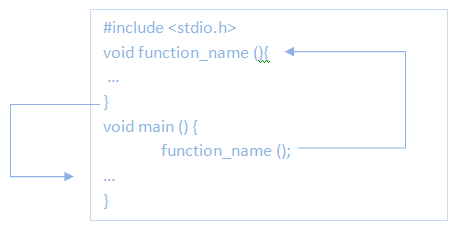From above diagram, it is clear how a function call works. It acts like a label, but it returns back to the calling block once its execution is over.

It increases the readability of the program as well as reduces the complexity of the program.

• If there is any repeating block of code, then if it is placed in a function and can be called wherever required. It makes the program simple and easy to understand.
• It uses a top down approach in the function – that means it performs all the high level logics of the function first then it moves to the lower level logic. To be even more specific, it first divides the problem into various tasks and creates functions for them. Later in the function it creates logic to solve those problems. For example, write a program to perform arithmetic operations like add / subtract/ multiply / divide the numbers. When we write a program, we see only add / subtract/ multiply / divide the numbers first and create respective function. More details of performing these operations – which is the lower levels of the operations, are performed within respective functions.
• We can re-use the functions in the same program or in other programs written by the same user. We can even create functions which can be re-used by other programs, other users or even in other systems.
• It helps in understanding the logic of the program and function well. Also, it makes the debugging the function easier than debugging a full length of single code. For example, if there is any error in division () function, then we can directly move to the function and fix the problem. But if there was no such function and a full length code was written, then we will end up in debugging full code. C allows us to compile and debug only the functions rather than compiling the whole program. This also an added advantage to debug the code. This makes testing easier.

## Function Declarations

User defined function will have to be coded by the user / developer and it has specific format that C compiler can understand. As a first step in creating a function, we have to declare them. It is equivalent to creating a memory space in the RAM to store the function and to perform the various tasks in the function.  A typical function has mainly two parts – function header and function body. A function header is the first line of the function which mentions the name of the function, arguments passed to the function and return type of the function. In short, a function header tells the structure of the function.

When we create a function in a program, we declare a function with its header. i.e.;

datatype function_name (arguments/parameters);

Here datatype is the datatype of the output of the function. It can be any primitive or non-primitive datatype. It can even be void – indicating that it is not returning any outputs. Next is the name of the function. Usually a meaningful name is given so that seeing the name itself one can understand what the function is doing. Name of the function should be unique to the program. No other function in the same program should have same function name. Next is the list of arguments / parameters. These are the variables with or without values passed to the function from the calling function. A function can be without any arguments too. If we pass the arguments, then we need to specify the datatypes of each of them.  We can pass any number of arguments to the function.

void fnDisplay (char chrString []); // a function with string parameter with no return values
int fnAddition (int intNum1, int intNum2); // a function with 2 integer arguments with integer output
float fnAverage (int intNum []); // a function with argument as integer array with return value as float

These are different function declaration depending on the requirement, input parameters and their return values. A function declaration is done before it is defined and used in the code. Usually we declare the function soon after the preprocessor directives.

``````#include <stdio.h>

//Function Declaration
void fnDisplay (char chrString []); // a function with string parameter with no return values
int fnAddition (int intNum1, int intNum2); // a function with 2 integer arguments with integer output
float fnAverage (int intNum []); // a function with argument as integer array with return value as float

void main (){
// example program
}``````

## Function Definitions

Function body is the set of code with statements and expressions using the parameters passed to it. It defines the functionality of the function. We can place function definition while declaring the function or anywhere in the program.

A typical function definition includes a function header followed by a open bracket – ‘{‘. Within the function body we can have declaration for local variables of the function, code to perform the tasks and a return statement to return the value to the calling function, followed by a close bracket – ‘}’.

``````datatype function_name (arguments/parameters) {
declaration part;
expressions/ statements;
return variable_name;
}
``````

This is the typical structure of a function body in C language. Below is the example program which shows how the control of the program jumps when a function is called. Here function definition is placed at the end of the code. But we have declared the function even before main function starts. When compiler compiles the code, it sees the declaration and understands that there is a definition for it. When we call the function in the main function, it replaces the function call with function definition and executes the code.  If we had not declared the function at the beginning and called it in the main function, compiler will not know that function is defined at the end and throw compilation error that function is not defined. We can even place the function definition while declaring itself.

``````#include <stdio.h>
void fnDisplay (char chrString []); // a function with string parameter with no return values

void main (){
char chrStr [] = "Example of a Function";
printf ("\nBefore calling the Function.....");
fnDisplay (chrStr); // calling the function
printf ("\nAfter calling the function.....");
}

// Function definition
void fnDisplay (char chrString []){
printf ("\nInside the function Body......\n");
printf ("%s", chrString);
printf ("\nEnd of the function Body.....");
}
``````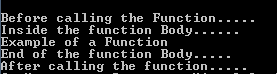## Calling Functions

We have learnt how to declare a function and define its definition. Now let us see how to call the function from another function.  A function can be called from any function / block of code by mentioning its name. In addition it should match the function definition – that means if a function has parameters passed to it, then we need to pass the parameters to the function by specifying them within brackets ‘()’. The type of the parameters should exactly match with the type that is declared in the function. Variable names passed to the function and the names specified in function declaration can be different. But the number of parameters and their type should always match.

fnDisplay (chrStr); // calling the function

Here the function accepts one argument and is of string type. While calling the function we are passing the same type of parameter. One may wonder why only the variable name is passed as parameter. Here string is an array of character and array acts like a pointer – when array name is specified it points to the first element of the array. Hence string is passed to function as any other normal variable. More details about the same is in pointer section. If we are passing any other variable type then we pass the value to the function parameter by specifying the variable name.

Here intVal1 and intVal2 are of integer type and when passed like above, its names are replaced by its value. Hence when this call is replaced by its definition, compiler gets the value at intNum1 andintNum2 respectively.

If a function is returning the value, then the function call should be assigned to a variable which is of the return type. In fnDisplay function, return type is void. Hence we need not assign function call to any variable. In fnAddition function, it returns the result to the calling function. Hence we have to capture the result in some variable with same type. Hence we call the function like below:

intResult = fnAddition (intVal1, intVal2); //calls the function

``````#include <stdio.h>

void main(){
int intVal1, intVal2, intResult;

scanf("%d", &intVal1);
scanf("%d", &intVal2);
intResult = fnAddition(intVal1, intVal2); //calls the function
printf("\nSum of two number is:%d", intResult);
}
// Function definition
int fnAddition (int intNum1, int intNum2){
return intNum1 + intNum2; // returns the sum of two numbers
}
``````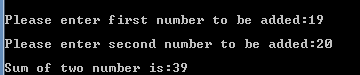## Function Parameters

We know what parameters of functions are. But how to pass the parameters to the function and how their values will be assigned to function parameters in its definition. The parameters declared in the function declaration are called formal parameters. They are created when function is called and acts as a local variable within the function. They are deleted from the memory as the function completes. It will be again created at some other memory location, if the same function is called again.

``````int fnAddition (int intNum1, int intNum2); // intNum1 and intNum2 are formal parameters
void fnDisplay (char chrString []){//chrString[] is formal parameter
printf ("\nInside the function Body......\n");
printf ("%s", chrString);
printf ("\nEnd of the function Body.....");
}``````

The parameters / variables passed to the function while calling the function is called as actual parameters of the function. They have the actual values to the function parameters and depending upon their value function is evaluated. In code names of the actual and formal parameters can be same or different. We keep it different to differentiate them.

fnDisplay (chrStr); // chrStr is the actual parameter
intResult = fnAddition (intVal1, intVal2); // intVal1 and intVal2 are the actual parameters

### There are two ways of passing the passing actual parameters to the function.

• Pass by value

In this method, when a function is called, actual parameters will have the actual values to be evaluated. When compiler replaces the function call by its definition, its formal parameters are replaced by the values. Since formal parameter gets the value to them, any changes to the formal parameter variable will not change the value of actual parameter variable. It may have affect within the function, but when returned to the calling function the actual parameter value will remain unchanged.

intResult = fnAddition (intVal1, intVal2); // intVal1 and intVal2 has the actual value of the parameters

when compiler compiles the code, it replaces the above function call like below:
Suppose intVal1 = 10 and intVal2 = 40, then

``````int fnAddition (10, 40){
return 10 + 40; // returns the sum of two numbers
}
``````

Here we have not modified the formal parameter and hence there are no changes to the values within the function. Hence there are no changes to the actual parameters too.

Suppose we have another function like below which simply increments the value of the parameter passed by 10. Suppose intVal has value 25.

intResult = fnIncrement (intVal); // intVal is the actual parameter

When the function is called like above compiler sees this as

intResult = fnIncrement (25); // intVal is the actual parameter

It replaces this call by function definition as below :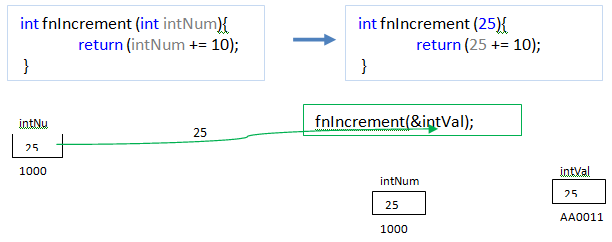We can see the same effect of actual and formal parameters in the below program. After the function, we can notice that the value of actual parameter is not changed even though the value of formal parameter is changed in the function. Even though formal parameter gets replaced by the value passed, it acts as a local variable within the function. Its value vanishes as soon as function completes.

``````#include <stdio.h>
int fnIncrement (intVal);

void main (){
int intVal=25, intResult;

printf ("\nValue of intVal before function call is %d", intVal);
intResult = fnIncrement(intVal); //calls the function
printf ("\nValue of intVal after function call is %d", intVal);
printf ("\nIncremented Value is:%d", intResult);
}
// Function definition
int fnIncrement (int intNum){
printf ("\nValue of intNum before incrementing is %d", intNum);
intNum += 10;
printf ("\nValue of intNum after incrementing is %d", intNum);
return intNum;
}``````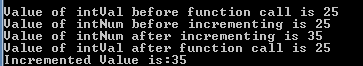• Pass by reference

In this method, we pass the address of the actual variable where the value to be passed is stored. That means reference to the value is passed which is not actual value of it. Hence here both formal and actual parameters will be pointing to the same memory locations in the memory. Thus any changes to formal or actual parameter will change both the values. This means address pointed by both the parameters remains same, but the value in that address can change to any value.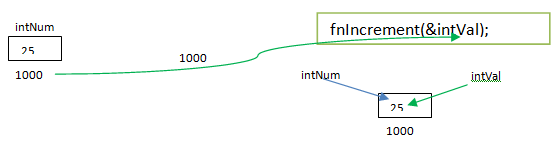Consider the same increment program above. Let us pass the parameter by reference – pass the address of the intVal as parameter when function is called. Then the formal parameter intNum also gets the address of the intVal and hence both refer to the same value now.

``````#include <stdio.h>
int fnIncrement (int *intNum); // formal parameter needs to be a pointer, inorder to accept the address

void main (){
int intVal = 25, intResult;

printf ("\nValue and Address of intVal before function call is %d and %x", intVal, &intVal);
intResult = fnIncrement (&intVal); //pass by reference
printf ("\nValue and Address of intVal after function call is %d and %x", intVal, &intVal);
printf ("\nIncremented Value and address of intResult is:%d and %x", intResult, &intResult);
}
// Function definition
int fnIncrement (int *intNum){// even though address is passed, the '*' now points to the value at the address passed
printf ("\nValue and Address of intNum before incrementing is %d and %x", *intNum, intNum);
*intNum += 10; // inorder to increment the value at the address passed, pointer notation needs to be used. Address of the variable remains same
printf ("\nValue and Address of intNum after incrementing is %d and %x", *intNum, intNum);
return *intNum; //returns the incremented value
}
``````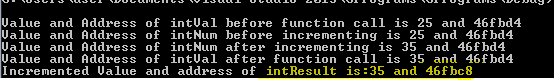In the program, we can notice that function is declared with pointer variable. The pointer variable is points to the address of another variable. Hence when we pass the address of actual parameter to the function call, formal parameter, intNum is created to point to intVal. In the function, when we increment intNum by 10, we are supposed to increment the value of intNum, but not the address. Hence we use ‘*’ before the intNum. Thus the address pointed by intNum remains unchanged even though value changes. After the increment intNum and intVal will have the same value and address. Since we have returned the value to the calling function (note here that we have not returned the address, but the value), intResult will get the value at different address. Suppose we have returned the address of intNum, then intResult would also have pointed to same address.

Below program shows how to get intResult also at the same address. All of these parameters use pointers to do this. It may look little complex at this stage, but it will be clear once pointer is understood. Always remember that *Num indicate the value at another address location, Num indicate address of other location and &Num is its own address. But please note how function is declared, called, how values are passed, returned and displayed.

``````#include <stdio.h>
int *fnIncrement (int *intNum); // formal parameter needs to be a pointer, inorder to accept the address

void main () {
int intVal = 25,*intResult;

printf ("\nValue and Address of intVal before function call is %d and %x", intVal, &intVal);
intResult = fnIncrement (&intVal); //pass by reference
printf ("\nValue and Address of intVal after function call is %d and %x", intVal, &intVal);
printf ("\nIncremented Value and address of intResult is:%d and %x", *intResult, intResult);
}
// Function definition
int *fnIncrement (int *intNum){// even though address is passed, the '*' now points to the value at the address passed
printf ("\nValue and Address of intNum before incrementing is %d and %x", *intNum, intNum);
*intNum += 10; // inorder to increment the value at the address passed, pointer notation needs to be used. Address of the variable remains same
printf ("\nValue and Address of intNum after incrementing is %d and %x", *intNum, intNum);
return intNum; //returns the incremented value
}``````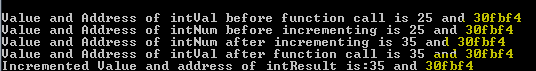These types of features of functions are mainly used when we have to copy two values, swap two numbers etc.

Sometimes, we might not know the number of parameters to be passed and their datatypes. In such situations, we can create functions with optional parameters. But we should pass at least one parameter with known datatype. Since the function accepts variable number of arguments / parameters, the function is called Variadic function. The general syntax for declaring such function is below:

datatype function_name (datatype argument1,…);

Here ‘…’ denotes the compiler that it has optional arguments of any type and any number. In the function, we address the optional parameters using a macros like va_start, va_list etc. These macros are defined in the header file stdarg.h and we have to include this file if we are using variadic functions. First argument in the function declaration and definition is compulsory. Usually this first argument would be the number of arguments passed to this function. When we call the function, we specify, how many arguments that we are going to pass, followed by the actual argument list. Thus at the run time, the function call gets to know how many arguments are being passed to it.

It uses some macros that are used to capture variable arguments passed, process each arguments etc.

• va_list : This is a library variable used to hold the arguments passed to the variadic function. We declare a variable of type va_list to capture the arguments passed to the function.

va_list variable_name;
va_list parameters; // declare a variable 'parameters' of type va_list

This library variable is suitable for holding the variables for the macros that are used by the variadic functions like va_start, va_end and va_arg.

• va_start : This is the macro used to initialize the variable list of parameters to the variable declared by va_list. This macro captures all the parameters passed to the function into va_list type of variable and points to the beginning of the argument list. This macro takes two arguments – one is the parameter list variable of type va_list and other one is the last argument which is the first fixed argument passed to the function (functions traverse the argument lists from last to first; hence the fixed first argument is called last argument).

va_start (va_list arg_list, last_arg);
va_start (parameters, intCount); //Starts accessing the optional parameter from the beginning

Here ‘parameters’ will be initialized to have the variable list of parameters passed to the function call. ‘intCount’ is the last argument of the function, which  is here,  a number of arguments passed to the function.

• va_arg : This macro is used to retrieve the next argument from the parameter list.
``     type va_arg(va_list arg_list, type));``

It traverses each argument in the argument list with its datatype as ‘type’. We will not know the number and datatype of the parameters passed to the function. Hence we need to assign some datatype to the arguments traversed and user explicitly defines the type for the arguments that are retrieved using va_arg macro. It returns a argument which is of same ‘type’ that va_arg macro has.

intParam=va_arg(parameters, int));// retrieves the parameters from ‘parameters’ and casts them to of type ‘int’ and assigns it to a variable ‘intParam’ which is also ‘int’ type.

• va_end : This macro is used to indicate the end of the usage of parameter list of the function. If this macro is not called, function will not be returned and its result will be undefined. Even though the macro va_end does not return anything, we need to specify this macro to indicate the end of the usage of the variable parameters of the function.

va_end(va_list arg_list); // end the use of parameters
va_end(parameters); // end the use of parameters

``````#include <stdarg.h>
#include <stdio.h>

// Function declaration and definition
int intSum = 0;
va_list parameters; // declare a variable 'parameters' of type va_list

va_start (parameters, intCount); //Starts accessing the optional parameter from the beginning
printf("\nNumber of parameters passed is:%d", intCount);

printf("\nNumbers that are passed to the function are:\n");
for (int index = 0; index < intCount; index++)
printf("%d\t", va_arg(parameters, int));

va_start(parameters, intCount); //Starts accessing the optional parameter from the beginning
for (int index = 0; index < intCount; index++)
intSum += va_arg(parameters, int);

va_end(parameters); // end the use of parameters
return intSum; //return the result
}

void main (){
int intResult;
intResult = fnAddition (5, 10, 20, 30, 40, 50); //calls the function 5 parameters
printf ("\nSum the numbers is:%d", intResult);
}
``````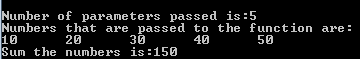## The main Function

This is the important function of any C program. Any program must have this default function in it. This function is the one from which execution of the C program begins. Hence we need not declare the prototype of the function, but we have to define this function. That means, we need to have this main function where we accepts the values, call the functions, display the results etc. The main function is usually has return type as int, which is used to indicate the status of the program to the compiler. If we use its return type as int, program should have ‘return 0;’ at the end to indicate program has been compiled successfully. But we can also have its return type as void – indicating no status to the compiler.

This function may or may not have arguments. Arguments are passed to the function when we need to capture the inputs through command line while executing the program. If the user enters the input while executing the program like above all examples, we need not pass the arguments to the main function.

``````#include <stdio.h>

int main (){
printf ("\Example of main function without arguments");

return 0; // indicates compiler that program is executed successfully and can exit from the program
}
``````

When arguments are passed to the main function, it accepts two arguments – one number of arguments with integer type and the other one is the array of parameters with char type. It can be named anything but they should have same datatype as explained.

int main (int argc, char *argv []) { ….}

argc – is the number of parameters to be passed to the function

*argv []– is the character pointer to the array of the arguments. It will accept argc number of parameters into its array.

``````#include <stdio.h>

int main(int argc, char *argv[]){

printf("\nTotal Number of arguments passed is : %d", argc);
printf("\nArguments passed through command line is : \n");
for (int index = 0; index<argc; index++)// traverses each arguments one by one
printf("%s\t" , argv[index]);

return 0; // indicates compiler that program is executed successfully and can exit from the program
}
``````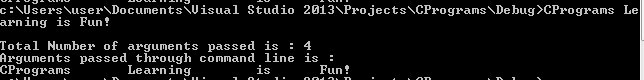## Recursive Functions

These are the functions which are called within the same function repeatedly. That means a function is called by itself.

``````datatype fn_name (){
….
fn_name(); // same function is being called
….
}
``````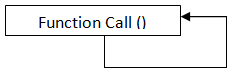The famous example for explaining recursion function is the factorial of a number. Factorial of a number is the product of the numbers and factorial of number -1. i.e.;
factorial (n) = n*factorial (n-1)
= n*(n-1) * factorial (n-2)
=    ….
= n* (n-1) * (n-2)*….. * 3*2* factorial(1)
= n* (n-1) * (n-2)*….. * 3*2* 1
Here we can observe the pattern in calculating the factorial of a number. That is it is repeatedly calculating the factorial of previous number and multiplying it with the current number. That means, when we write a function to calculate the factorial, we need not write any for loop or while loop where we continuously multiply the numbers to get the factorial. Instead we can repeatedly call the same function itself till we get number 1. In short, a factorial function will be like below :

``````int fnFactorial(int intNum){
if (intNum < 1)
return 1;
else
return (intNum * fnFactorial(intNum - 1));
}
``````

Since the function is called within the same function, we need to be careful to create recursive function. If proper stopping command is not used, it may end up in infinite loop. In above example, there is a condition to check if the number is less than one. If it is less than one, then we are not calling the function there; instead returning 1 by stopping the function call. But if the number is greater than or equal to one, we are continuously calling the function for the number equal to intNum -1. This is how it recurses the function and stops at one point.

``````#include <stdio.h>
int fnFactorial(int intNum);

void main(){
int intVal;

printf("\n Please enter the number whose factorial to be found:");
scanf("%d", &intVal);
printf("\n Factorial of a number %d is : %d:", intVal, fnFactorial(intVal));
}
int fnFactorial(int intNum){
if (intNum < 1)
return 1;
else
return (intNum * fnFactorial(intNum - 1));
}
``````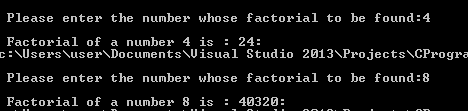## Static Functions

Suppose we have to write some functions which are internal to the program, and we do not want any users to use that function. These internal functions may be used by some other function in the same program but should not be accessible to other programs, files or users. For example,  suppose we have a program where we generate various reports like student grade report, college annual report, and some other staff reports. But all these have a common report header with college name, address, phone, fax etc. Hence we can have a function to have all these header information to be printed on the report. But function for displaying header will be called by the different functions which generate reports. Hence this header function has nothing to do with users. Similarly other programs or related to student do not require  these header function. In short, we do not want any other programs or files or users to access this header function which is specifically created for having report header. Thus we can hide this header function from any other files or user and make it to be used by the file/program in which it is written. This is done by using a keyword ‘static’ before the function declaration.

static datatype function_name (argument/s);

A static function is the one which can be accessed by the functions in the file in which it is created. It is not publically available for the users or other programs.

Let us create two files - std_staff_report.c to display student, staff and annual reports and displayHeader.c file to display header of the report.  Let us call displayHeader() function in the displayHeader file from std_staff_report.c file without making the function as static. It will work normally as any other file. If we make the function as static, the function call from std_staff_report.c file will throw an error. If we write those functions in std_staff_report.c within displayHeader.c file and call them it will work normally. That means, static functions are available to only those functions that are all in the same file. Static functions are not available to any other user / file/ program other than where it has been written.

``````// displayHeader.c
#include <stdio.h>
}
``````

``````//std_staff_report.c
#include <stdio.h>
#include "displayHeader.c"// comment this line to see the affect of static
void displayStdReport(char *stdName);
void displayStaffReport(char *staffName);
void displayAnnualReport();

void main(){
printf("\n\nStudent Report is:");
displayStdReport("Rose");
printf("\n\nStaff Report is:");
displayStaffReport("Robert");
printf("\n\nAnual Report is:");
displayAnnualReport();

}
void displayStdReport(char *stdName){
printf("\nInside %s Student Report Function:", stdName);
printf("\nDisplaying %s Student Report :", stdName);

}
void displayStaffReport(char * staffName){
printf("\nInside %s Staff Report Function:", staffName);
printf("\nDisplaying %s Staff Report:", staffName);
}
void displayAnnualReport(){
printf("\nInside Annual Report");
printf("\nDisplaying Annual Report");
}
``````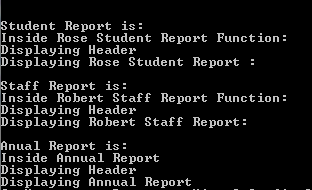## Nested Functions

Nested functions are the functions that have one or more functions being called within it. For example, a function call within a main function makes main function as a nested function. There is no limit on number of functions being nested. We have already seen nesting of functions in main function, recursion function, static function etc.

``````datatype function_name(arguments){
datatype function_name1(); // declare another function
…
datatype function_name1(); // call the function
….
datatype function_name1(){ //define the function
…
}
}``````

Different arithmetic operations performed within the main function is an example of nested funtion.

``````#include <stdio.h>

//Function Declaration
void minus(int intNum1, int intNum2);
void divide(int intNum1, int intNum2);
void multiply(int intNum1, int intNum2);

void main(){
// calling different functions within another function
minus(100, 23);
divide(25, 5);
multiply(400, 7);
printf("\n End of the arithmetic Operation….");
}

void add (int intNum1, int intNum2){
printf("\nSum of %d and %d is : %d", intNum1, intNum2, intNum1 + intNum2);
}
void minus(int intNum1, int intNum2){
printf("\nDifference of %d and %d is : %d", intNum1, intNum2, intNum1 - intNum2);
}
void divide(int intNum1, int intNum2){
printf("\nResult of %d / %d is : %d", intNum1, intNum2, intNum1 / intNum2);
}
void multiply(int intNum1, int intNum2){
printf("\nResult of %d * %d is : %d", intNum1, intNum2, intNum1*intNum2);
}
``````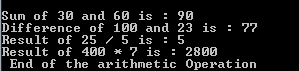## Summary

• A function is a named reusable block of code in the program.
• There are two types of functions – Library Functions and User Defined Functions.
• Library functions are – main(), scanf, gets, getchar, printf, puts, putchar, malloc, calloc, sqrt, sin, cos, tan, floor, exp, tolower, toupper, isdigit, isalpha etc.
• Parameters to the function can be passed in two ways – pass by value and pass by reference.
• In pass by value, value of the actual parameter is passed and function cannot change the value of actual parameter
• In pass by reference, address of the actual parameter is passed and any changes to the value of the formal parameter will change the value of actual parameters too. But address of both the parameter remains the same.
• Variadic functions are the functions which accept variable number of parameter of any type.
• Static functions are functions which are not visible to the files or programs or users other than the file where it has been written.
• Nested functions are the functions defined within another functions.
• Recursive function is the one in which same functions are repeatedly called within it.

Scroll to Top